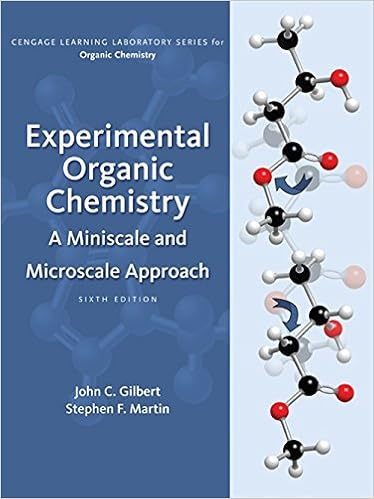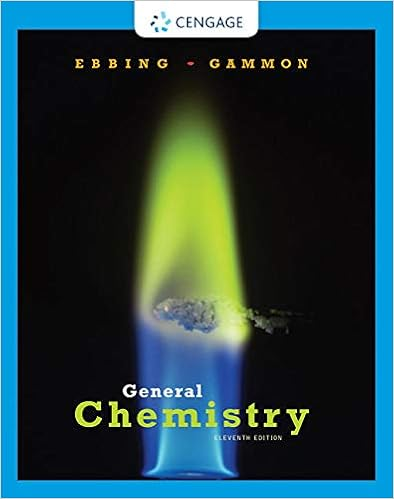# Lab 9 Determination of the Dissociation Constant of a Weak Acid (2).docx

• Lab Report
• 10
• 67% (3) 2 out of 3 people found this document helpful

This preview shows page 1 - 3 out of 10 pages.

1Lab 9Determination of the Dissociation Constant of a Weak AcidUSF-SM, General Chemistry II LaboratoryTo become familiar with the operation of a pH meter and use pH to determine the magnitude ofthe equilibrium constant of a weak acid.ApparatuspH meter with electrodes250 mL beakers (2)100 mL beakers (2)Burette25 mL pipet and pipet bulbBurette clamp and ring standRing stand and ringRO water bottleChemicalsStandard buffer solution (pH = 4.00 and pH = 7.00 Buffer)Unknown solution of a weak acid0.1 M NaOHIntroductionAcid - Base EquilibriaAccording to the Brnsted- Lowry acid- base theory, the strength of an acid is related to its abilityto donate protons. All acid- base reactions are then competitions between bases of variousstrengths for these protons. For example, the strong acid HCl reacts with water according toEquation .HCl(aq) + H2O(l)H3O+(aq) + Cl-(aq) {1}This acid is a strong acid and is completely dissociated, in other words, 100% dissociated in diluteaqueous solution. Consequently, the [H3O+] concentration of 0.1MHCl is 0.1M.By contrast, acetic acid, CH3COOH (abbreviated HOAc), is a weak acid and is only slightlydissociated to form hydronium and acetate (abbreviated OAc-) ions, as shown in equation {2}.H2O(l) + HOAc(aq)H3O+(aq) + OAc-(aq) {2}Therefore, its acid dissociation constant, as shown by Equation {3}, is small.K=[?3?+][???]=1.8×105{3}a[????]Acetic acid only partially dissociates in aqueous solution, an appreciable quantity of undissociatedacetic acid remains in solution.
##### We have textbook solutions for you!The document you are viewing contains questions related to this textbook.
Chapter 20 / Exercise 03
Experimental Organic Chemistry: A Miniscale & Microscale Approach
Gilbert/MartinExpert VerifiedBrowse all Textbook Solutions
For the general weak acid HA in aqueous solution, the dissociation reaction and dissociationconstant expression are,HA(aq) + H2O(l)H3O+(aq) + A-(aq) {4}K =[?3?+][?]{5}a][??Recall that pH is defined as pH = -log [H3O+] {6}Solving Equation {5} for [H3O+] and substituting this quantity into equation {6} yield[H3O+]= K][??{7}][?-log [H3O+] = -logKa-log][??[?]{8}pH = pKa-log][??

Course Hero member to access this document

Course Hero member to access this document

End of preview. Want to read all 10 pages?

Course Hero member to access this document

Term
Spring
Professor
Dr.LauraAnderson
Tags
##### We have textbook solutions for you!
The document you are viewing contains questions related to this textbook.The document you are viewing contains questions related to this textbook.
Chapter 20 / Exercise 03
Experimental Organic Chemistry: A Miniscale & Microscale Approach
Gilbert/MartinExpert Verified
•••